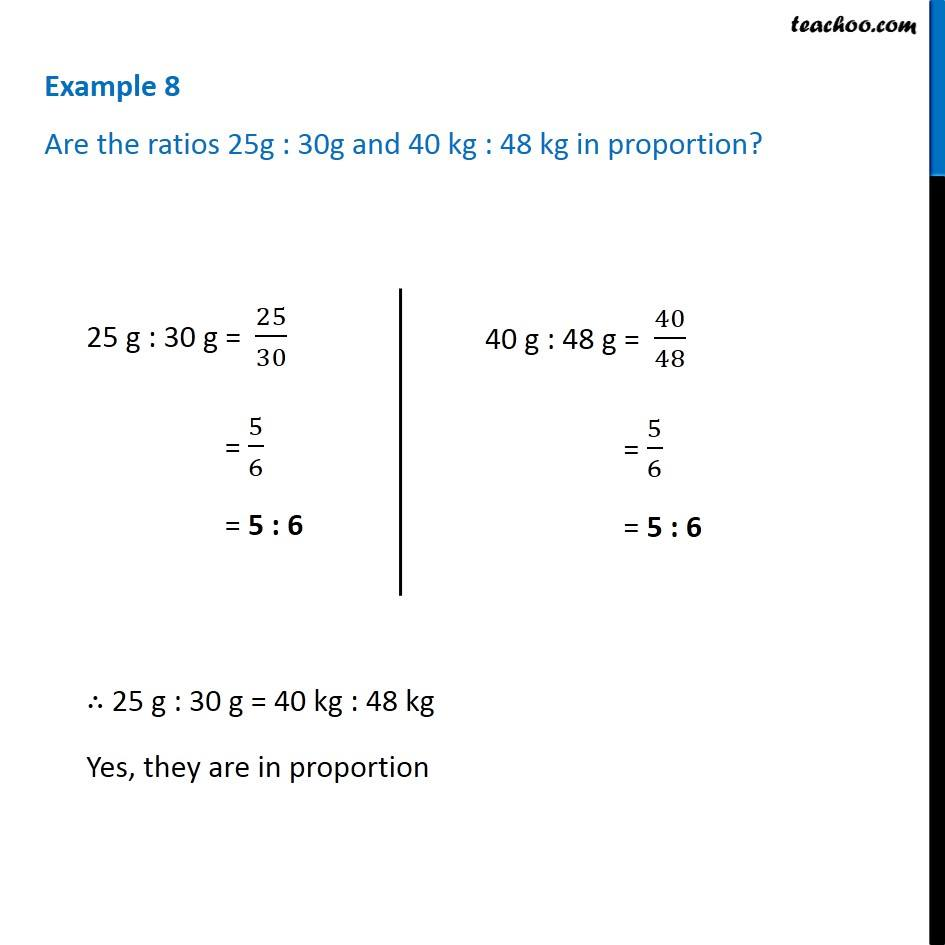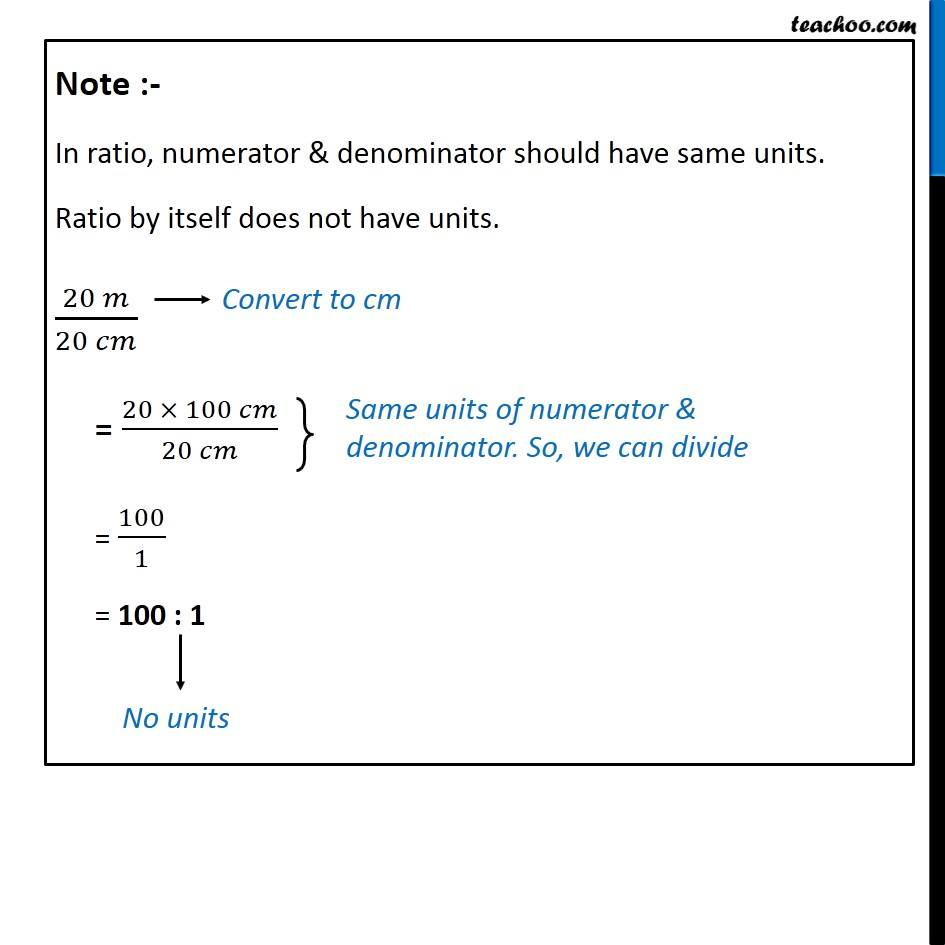Proportion

Chapter 12 Class 6 Ratio and Proportion
Concept wiseLearn in your speed, with individual attention - Teachoo Maths 1-on-1 Class

### Transcript

Example 8 Are the ratios 25g : 30g and 40 kg : 48 kg in proportion?25 g : 30 g = 25/30 = 5/6 = 5 : 6 40 g : 48 g = 40/48 = 5/6 = 5 : 6 ∴ 25 g : 30 g = 40 kg : 48 kg Yes, they are in proportion Note :- In ratio, numerator & denominator should have same units. Ratio by itself does not have units. (20 𝑚)/(20 𝑐𝑚) = (20 × 100 𝑐𝑚)/(20 𝑐𝑚) = 100/1 = 100 : 1